#### You may also likeMake a set of numbers that use all the digits from 1 to 9, once and once only. Add them up. The result is divisible by 9. Add each of the digits in the new number. What is their sum? Now try some other possibilities for yourself!### Counting Factors

Is there an efficient way to work out how many factors a large number has?### Repeaters

Choose any 3 digits and make a 6 digit number by repeating the 3 digits in the same order (e.g. 594594). Explain why whatever digits you choose the number will always be divisible by 7, 11 and 13.

# Legs Eleven

##### Age 11 to 14Challenge Level

Lots of people tried out numbers and found that the result was divisible by 11 for 4 digit numbers, and some people tried numbers of other lengths.

 Name Number Sum 11 times table Geon 3412 3412 + 4123 7535 = 685$\times$11 Jun 1000 1000 + 0001 1001 = 91$\times$11 Mahnoor 3621 2163 3621 + 6213 2163 + 1632 9834 = 894$\times$11 3795 = 345$\times$11 Yusuf, Shafi, Adam 97685437 97685437 + 76854379 174539816 = 15867256$\times$11 Helen 34 826 34 + 43 826 + 268 77 = 7$\times$11 1094 is not divisible by 11 Nabiha 38 5463 832596 38 + 83 5463 + 4635 832596 + 325968 121 = 11$\times$11 10098 = 918$\times$11 1158564 = 105324$\times$11 Aadit, Nicole, Jedi 6953 3484 9876 3527 4567 1000 6953 + 9536 3483 + 4843 9876 + 8769 3527 + 5273 4567 + 5674 1000 + 1 16489 = 1499$\times$11 8827 = 757$\times$11 18645 = 1695$\times$11 8800 = 800$\times$11 10241 = 931$\times$11 1001 = 91$\times$11

As a result of this, Geon from British International School of Hanoi in Vietnam, Nabiha, Helen, Victor, Peter and Edric and Yusuf, Shafi and Adam from Greenacre Public School in Australia and Darcy and Travis from St Stephen's School thought that numbers with an even number of digits would give a sum that was divisible by 11, but numbers with an odd number of digits would not.

Jordan, Ryan, Stephen, Nathan, Juliana and Presley from Monarch Global Academy sent in a solution that gives an insight into why it works for 4 digit numbers:
We agree that all whole four-digit numbers will add to a sum that is divisible by 11. Decimals will not.

For example
1,234
+2,341

The way I found my answer was splitting my numbers into expanded form:
1$\times$1,000 + 2$\times$100 + 3$\times$10 + 4$\times$1
2$\times$1,000 + 3$\times$100 + 4$\times$10 + 1$\times$1

Then I combined the numbers with the same digit, to make multiples of 11:
1,000+1 = 1,001;  200+2,000 = 2,200;     300+30 = 330;  4+40 = 44

All of these sums are multiples of 11, so their sum will also be a multiple of 11. In this situation, the answer was 3,575, which in the end was a multiple of 11.

All of the other examples we tried were too.

You will always have at least two of the same digit in each problem because you are just moving the digits in the original number around.

Thomas, Nathan, Max from Longsands Academy used the same method, but with letters to represent the digits:
First of all, we tried to disprove the statement given. We found out that it was true. After we decided that that it was true we tried symbolising the digits as letters. Starting off with ABCD. We thought about place value that the numbers held and once the place value had been shifted. It looked like this:
ABCD
+BCDA

Place value of letters are:
A=1000's and 1's
B=100's and 1000's
C=10's and 100's
D=1's and 10's

Therefore,
The A will be a multiple of 1001
The B will be a multiple of 1100
The C will be a multiple of 110
The D will be a multiple of 11

Because 1001, 1100, 110 and 11 are all multiples of 11, it does not matter what numbers are being used, as the sum will always be divisible by 11.

Anas from England, Thiago from Unicamp in Brazil, Marcus from Richard Hale, Thomas from Hollinwood Academy, Por Pan from British International School Phuket, Aadit, Nicole and Jedi from Mr McDonagh's math group at Bangkok Patana School in Thailand, and Victoria used a more formal algebraic method.

Victoria made a very clear video, which you can watch here. This is Anas' written work, which also includes 3, 5 and 6 digit numbers:

Let '$abcd$' represent the four digit number. For a four digit number, there is a 'thousands' column, a 'hundreds' column, a 'tens' column and a 'units' column. As the '$a$' is in the 'thousands' column, its actual value it $1000a$.
The '$b$' is in the 'hundreds' column, therefore its actual value is $100b$.
Similarly, the '$c$' is in the 'tens' column and therefore its real value is $10c$.
As the '$d$' is in the 'units' column, its value is just $d$.
So, writing out '$abcd$' algebraically, we get: $1000a+100b+10c+d$

Now, as we add on the number '$bcda$' (as we have to move the numbers from '$abcd$' to the left and therefore the a comes to the 'units' column), we'll do the same as we did for '$abcd$' for '$bcda$': $1000b+100c+10d+a$
We can now add the two algebraic expressions for the integers '$abcd$' and '$bcda$' by collecting like-terms and we'll get:$$(1000a+100b+10c+d)+(1000b+100c+10d+a)\\ =1001a+1100b+110c+11d$$
We can now factor out '$1001a+1100b+110c+11d$' and we'll get:$$11(91a+100b+10c+d)$$
âˆ´The sum of the two integers '$abcd$' and '$bcda$' is a multiple of $11$ always.

As for the three digit number case, let '$abc$' denote the three digit number. If we write '$abc$' algebraically, we get: $100a+10b+c$
Now writing an algebraic expression  for '$bca$', we get: $100b+10c+a$
We now add the two algebraic expressions together to get:$$(100a+10b+c)+(100b+10c+a) =101a+110b+11c$$
This, however, can't be factored out using $11$ and therefore the answer of the sum of '$abc$' and '$bca$' is not a multiple of eleven.

I will now do the same as has been done above for the previous two examples, except for a five digit number. Let '$abcde$' denote a five digit number. Writing '$abcde$' algebraically, we get: $10000a+1000b+100c+10d+e$
Now, writing out '$bcdea$' algebraically, we will get: $10000b+1000c+100d+10e+a$
Adding the two five-digit integers, we get:$$(10000a+1000b+100c+10d+e)+(10000b+1000c+100d+10e+a)\\ =10001a+11000b+1100c+110d+11e$$
The sum of the integers '$abcde$' and '$bcdea$' can't be factored using $11$ and therefore,  the sum of the integers '$abcde$' and '$bcdea$' is not a multiple of eleven.

For the case of a six digit integer, we'll let '$abcdef$' denote the integer. Writing the integer '$abcdef$' algebraically, we get: $100000a+10000b+1000c+100d+10e+f$
Writing the integer '$bcdefa$' algebraically, we get: $100000b+10000c+1000d+100e+10f+a$
Adding '$abcdef$' and '$bcdefa$' together, we get: $$(100000a+10000b+1000c+100d+10e+f)+(100000b+10000c+1000d+100e+10f+a)\\ =100001a+110000b+11000c+1100d+110e+11f$$
This can be factorised to give us: $$11(9091a+10000b+1000c+100d+10e+f)$$
So, the sum of '$abcdef$' and '$bcdefa$' is a multiple of eleven.

I did some other digit-numbers in my own time and I came to conclude that if you're doing the sum for numbers with an even amount of digits, then the answer will be a multiple of eleven. If the numbers have an odd number of digits, then the answer will not be divisible by 11. Therefore, if you used a 38-digit-number, the sum would be divisible by 11.

To show that it is true that this works only for numbers with even numbers of digits, Anny from St Mary's School Cambridge, Oak and Tiffany from British International School Phuket, Tom from King Edward Five Ways and Jack from Ashmole Academy in England looked at the coefficient of $a$. Tom and Jack used formal algebra and a divisibility test for 11 to prove the result. This is Jack's work:
With regards to a general solution for an $n$ digit number, you can, using my previous logic, state that the digits of the number are $a, b, c, d, ..., y, z$ in order.
(Actually, since there are 26 letters in the alphabet, this suggests a 26 digit number, so most mathematicians use $a_1, a_1, a_1, ...a_n$ instead of $a, b, c, ..., z$. However, here we can imagine that there could be any number of letters in the alphabet, as Jack has)
You can also write the number as an expression, in the form: $$10^{n-1}\times a + 10^{n-2}\times b + 10^{n-3}\times c + 10^{n-4}\times d + ... + 10y + z$$
The permutation can also be written in this form as:$$10^{n-1}\times b + 10^{n-2}\times c + 10^{n-3}\times d + 10^{n-4}\times e + ... + 10z + a$$
(by just shifting all of the digits to the left)

By adding these two expressions together and then simplifying, we get the expression:$$a\left(10^{n-1} + 1\right) +b\left(10^{n-2} + 10^{n-1}\right) + c\left(10^{n-3} + 10^{n-2}\right) + ... +z(10 + 1)$$
Now, let us take the expression of $10^{n-2} + 10^{n-1}$. If we express this in a numerical form, we will get the number $11$ followed by $(n-2)$ zeros, as $10^{n-2}$ is just $1$ followed by $(n-2)$ zeros and $10^{n-1}$ is just $1$ followed by $(n-1)$ zeros. Thus we can express $10^{n-2} + 10^{n-1}$ as $11 \times 10^{n-2}.$

In fact, we can do this with every part of the expression above, and can simplify yet again to: $$a\left(10^{n-1} + 1\right) + b\left(11\times10^{n-2}\right) + c\left(11\times10^{n-3}\right) ... + z(11\times1)$$
Or, factoring out the 11: $$a\left(10^{n-1} + 1\right) + 11\left(10^{n-2}b + 10^{n-3}c + ... + z \right)$$
So, as we wish $11$ to go into all of the above expression, all we need to do is prove $10^{n-1} + 1$ is divisible by $11.$ If we were to write this number out, it would be in the form $1$ followed by $(n-2)$ zeros followed by a $1.$ The divisibility rule for $11$ is to take the alternating sum of the digits in the number, read from left to right. If that is divisible by $11$, so is the original number. There are $2$ potential cases here: the digits sum to $0$ or to $2,$ as we can either have the expression $1 - 0 + 0 - 0 ... + 0 - 1 = 0$ OR $1 - 0 + 0 - 0 ... - 0 + 1 = 2.$

As $0$ is a multiple of $11$ ($11\times0$) but $2$ is not, we need to only find cases where $0$ occurs. This ONLY happens when $n$ is even, as when $n$ is even, the last alternating sign HAS to be a $-$. Thus, $10^{n-1} + 1$ is divisible by $11$ iff (IF AND ONLY IF) $n$ is even!

Thus, as two multiples of $11$ sum to a multiple of $11$, we can prove that for an $n$ digit number, WHEN $n$ IS EVEN, if you add the original number to its permutation you will always get a multiple of $11.$ Finally, QED

Tom used a different divisibility test which is described in this article to prove the claim that $10^{n-1}$ is divisible by $11$ only when $n$ is even:
From the NRICH page on divisibility tests, odd powers of $10$ are one less than a multiple of $11$ and even powers of $10$ are one more than a multiple of $11$. So in order for $10^{n-1}+1$ to be a multiple of $11$, $10^{n-1}$ must be one less than a multiple of 11, ${n-1}$ must be odd and therefore $n$ must be even.

Jamie from Bournemouth School in the UK used proof by induction to prove that $10^{n-1}$ is divisible by $11$ when $n$ is even. Jamie almost proves that it is never divisible by $11$ when $n$ is odd - the missing part is explained below.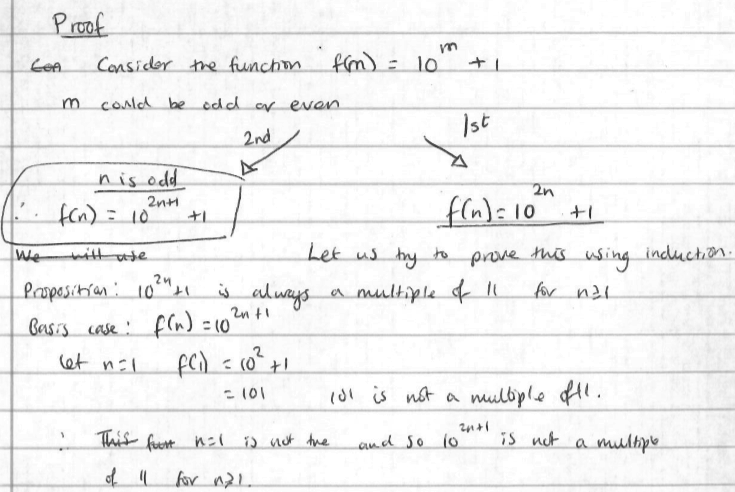In fact, this only proves that for $n=1$, $10^{2n}+1$ is not divisible by $11$ - it doesn't prove that it is not divisible by $11$ for larger values of $n$. However, the work needed to prove this is included later in the proof.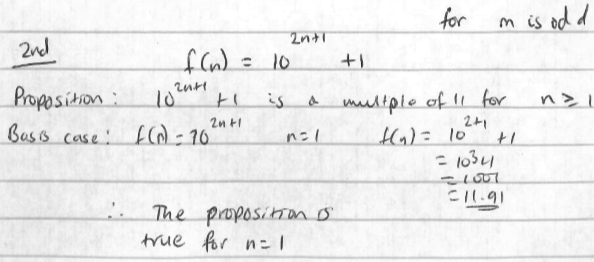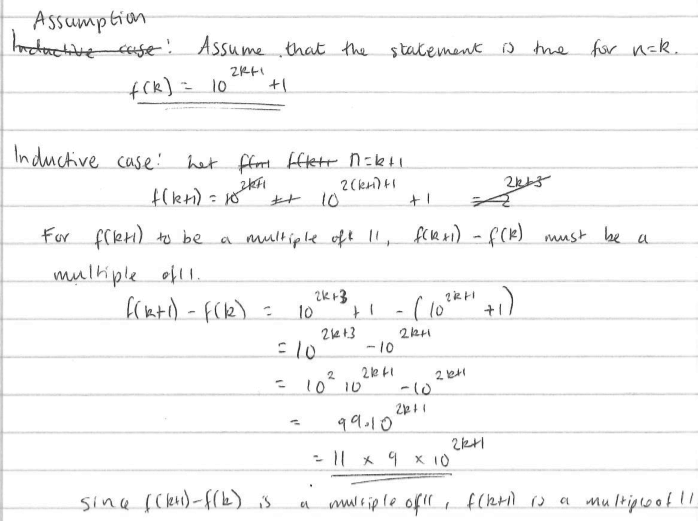Here, this same algebra would show that $\left(10^{2k+2}+1\right)-\left(10^{2k}+1\right)$ is a multiple of $11$, so for the even number case, $f(n+1)$ is divisible by $11$ if and only if $f(n)$ is - and given that, in this case, $f(1)$ is not, $f(2), f(3), ..., f(n)$ won't be either.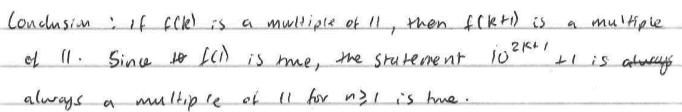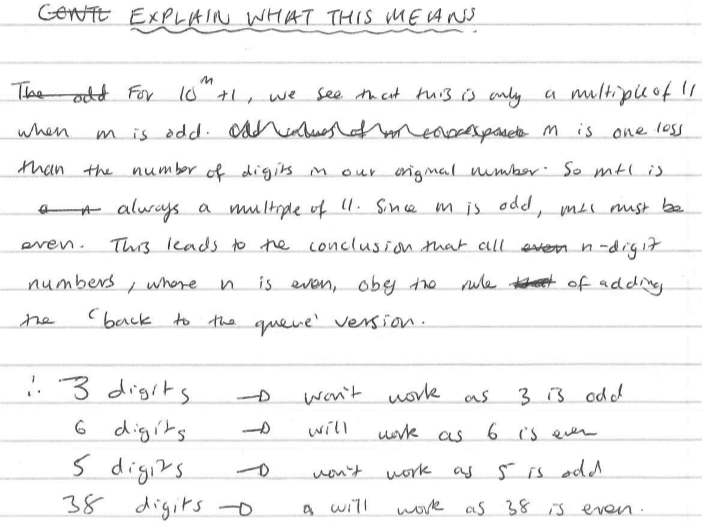Navjot from Sherborne Qatar wanted to prove that $10^n+1$ is divisible by $11$ if and only if $n$ is odd, and began a very brave proof using logarithms. Kevin also bravely attempted to prove the 4 digit case (that rearranging the digits and adding gives a multiple of 11) by induction. Both proofs include very good work, but both have a flaw.
Click here to see Navjot's proof with the flaw explained
Click here to see Kevin's proof with the flaw explained
Well done to both of you for following your curiosity and applying different techniques to create a proof.

Another way to determine when $10^n+1$ (or any other number) is divisible by $11$ it is by using modular arithmetic, which is introduced in this article. To do this, you need to read up to (and including) the section on multiplication, and then try writing the powers of 10 in modulo 11. (You can also use this technique to find out how Jack's divisibility test works).